# Bar graph with overlapping dots (Bar-dot) plot

What is the Bar-dot plot?

• This is a regular bar graph of the mean of data of each variable with overlapping dots for individual observation for that variable
• A good way to represent the mean of the variable with standard error bar and distribution of each observation

How to create Bar-dot plot?

• We will use `bioinfokit v0.6` or later
• Check bioinfokit documentation for installation and documentation

Useful reading: Data handling using pandas

``````# you can use interactive python interpreter, jupyter notebook, spyder or python code
# I am using interactive python interpreter (Python 3.7)
>>> from bioinfokit import analys, visuz
# load dataset as pandas dataframe
# data should not have missing values (NaN)
>>> df = analys.get_data('bdot').data
A   B   C   D
0  25  45  30  60
1  30  55  36  60
2  28  45  35  65
3  36  56  37  62
4  29  40  48  73

# create bardot plot with default parameters
>>> visuz.stat.bardot(df=df)
# set parameter show=True, if you want view the image instead of saving
# visuz.stat.bardot(df=df, show= True)
``````

Generated Bar-dot plot,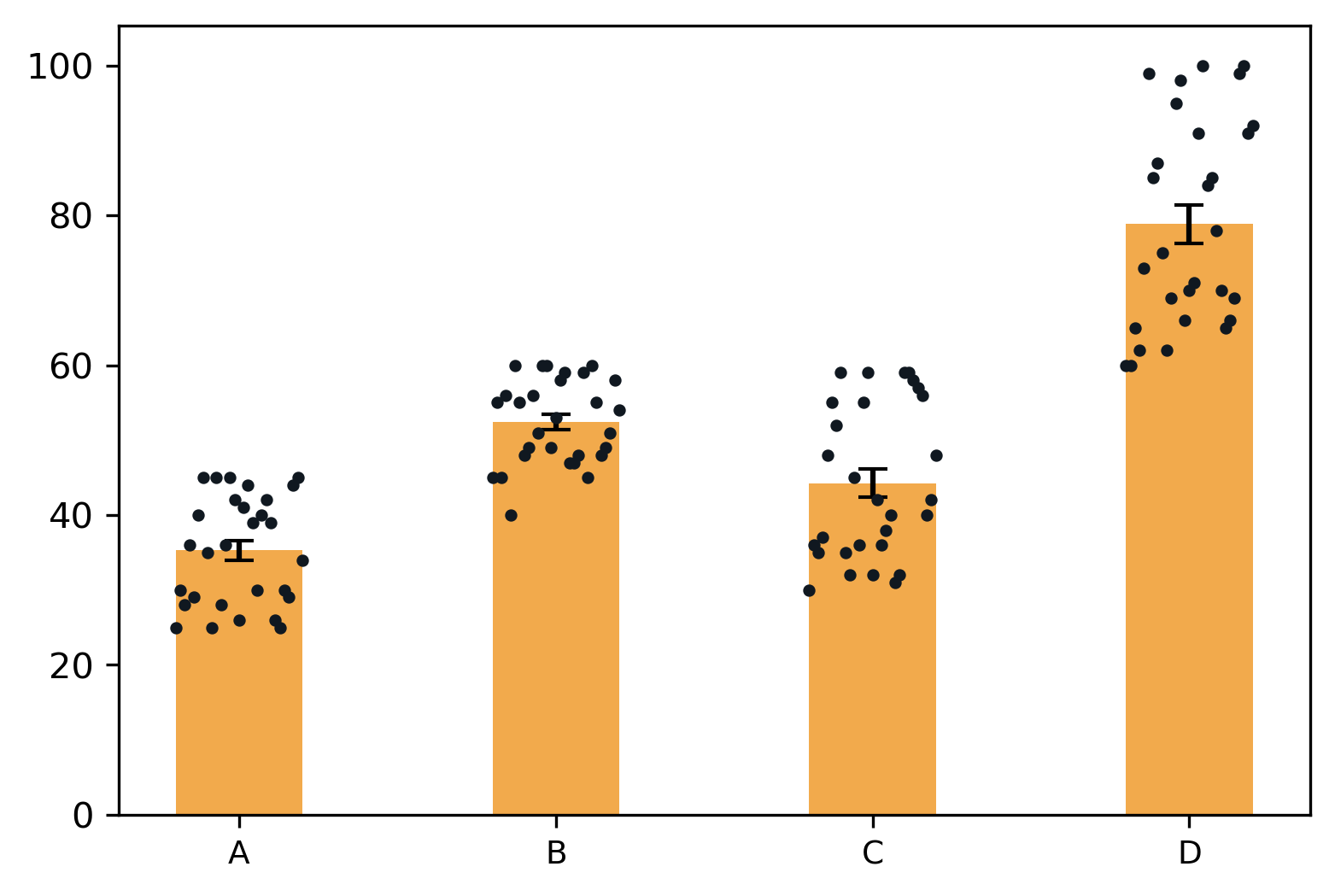Change bar colors

``````# add different color to each bar
>>> visuz.stat.bardot(df=df, colorbar=("#f2aa4cff","#e69a8dff", "#adefd1ff", "#5b84b1ff") )
``````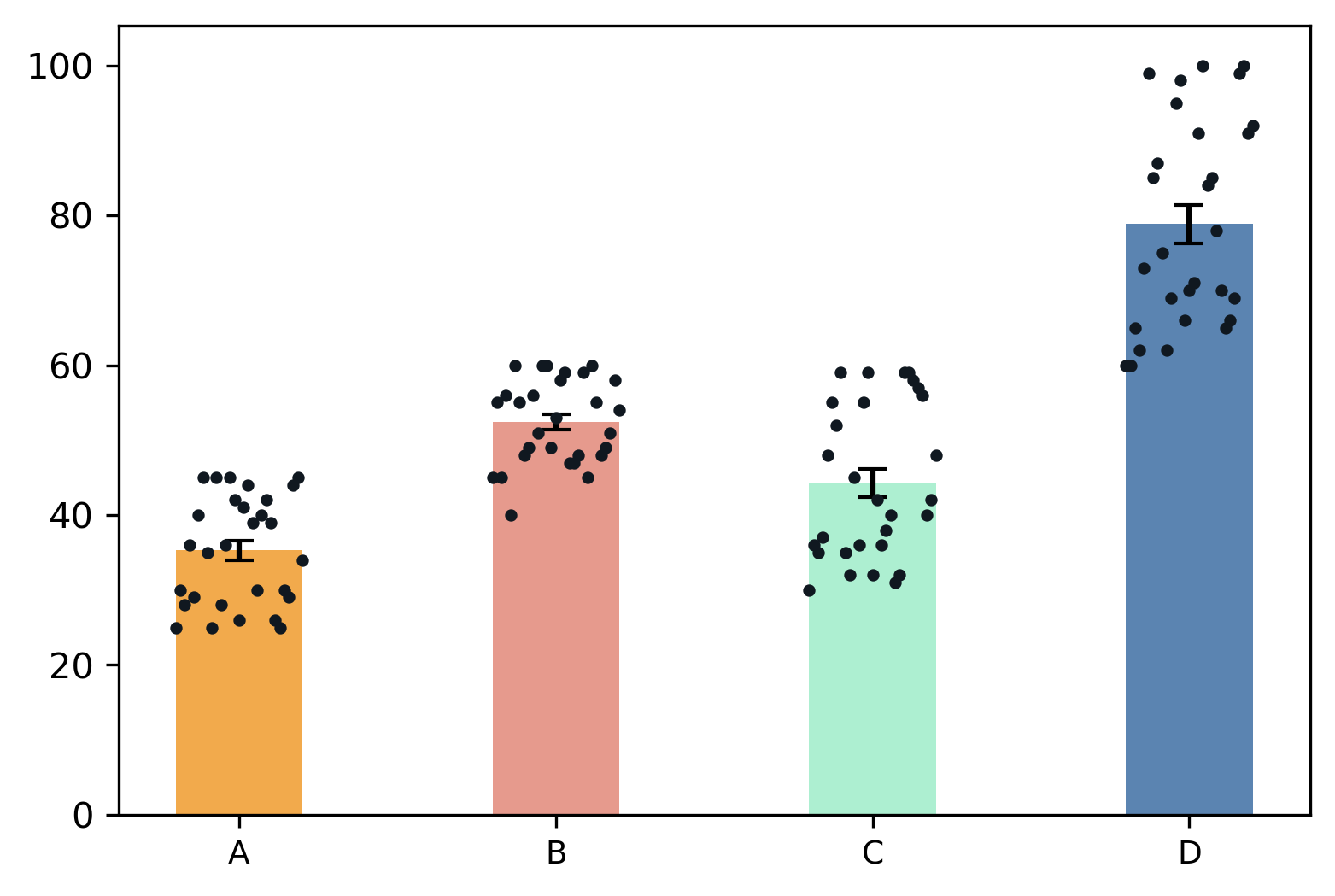Change dot colors

``````# change dot color for all bars
>>> visuz.stat.bardot(df=df, colordot=["#616247ff"])
``````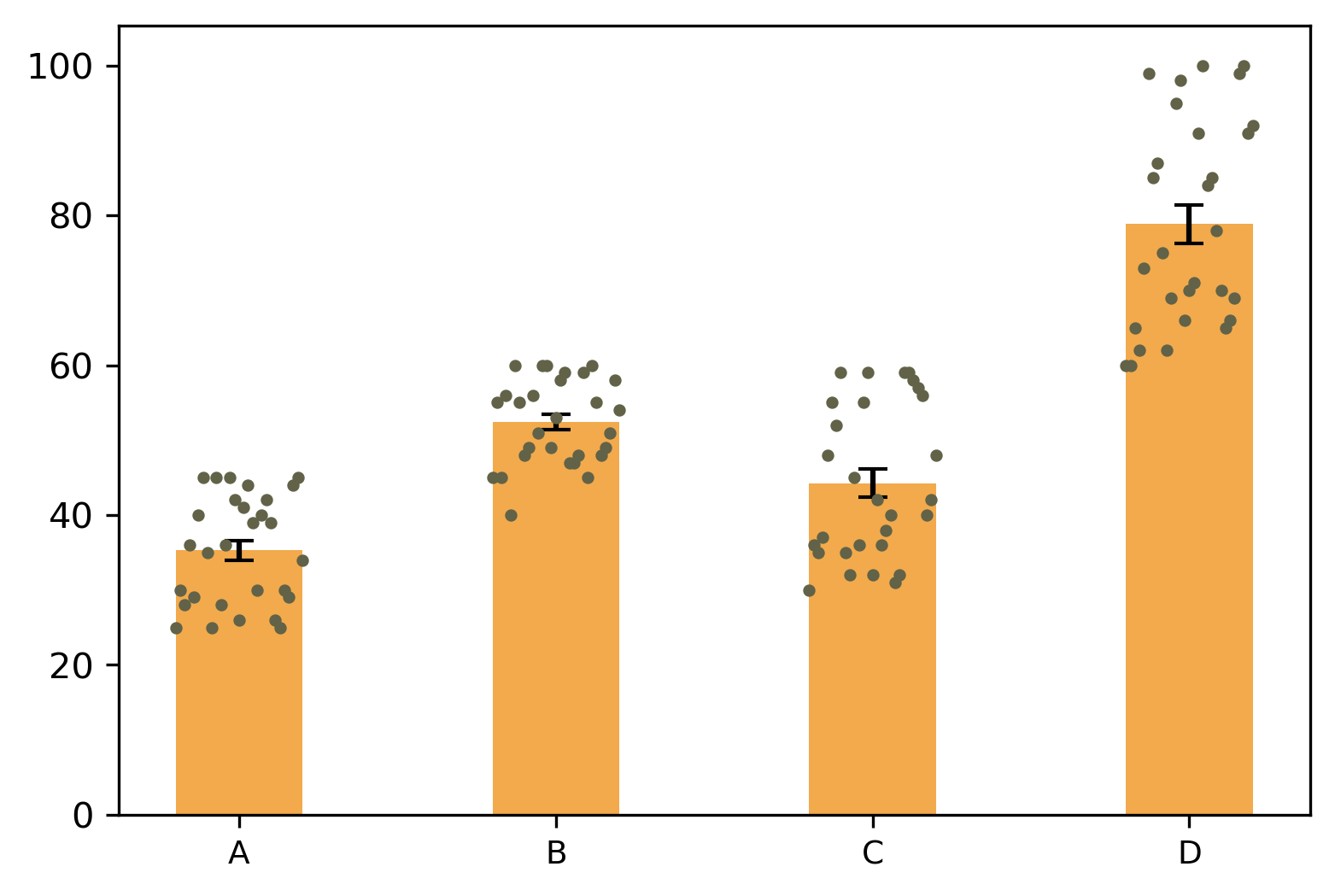``````# change dot color for each bar
>>> visuz.stat.bardot(df=df, colordot=["#616247ff", "#101820ff", "#006b38ff", "#3c1053ff"]  )
``````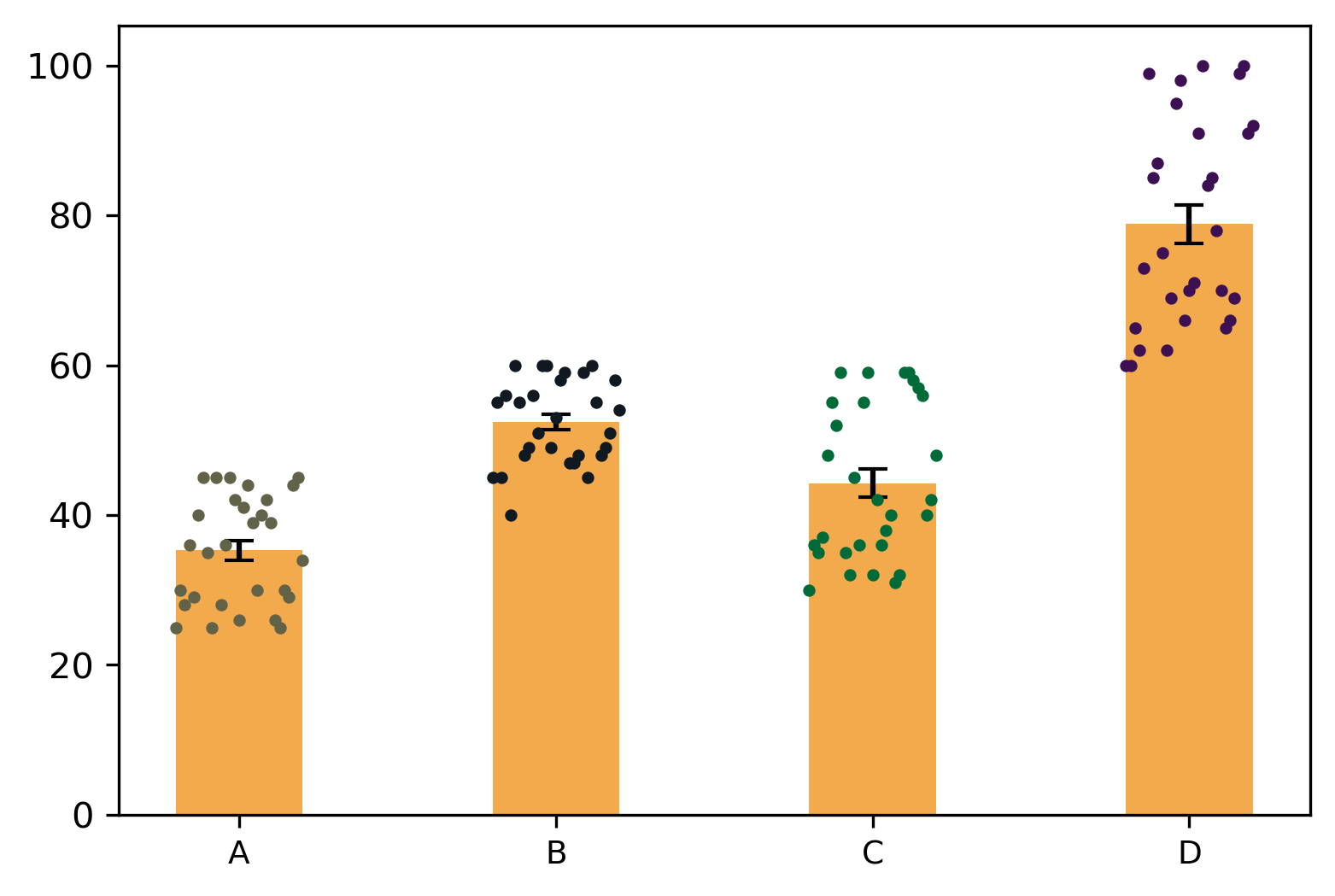Change shape of dots

``````# change shape of dot
# see available shapes at https://matplotlib.org/3.1.1/api/markers_api.html
>>> visuz.stat.bardot(df=df, markerdot="D")
``````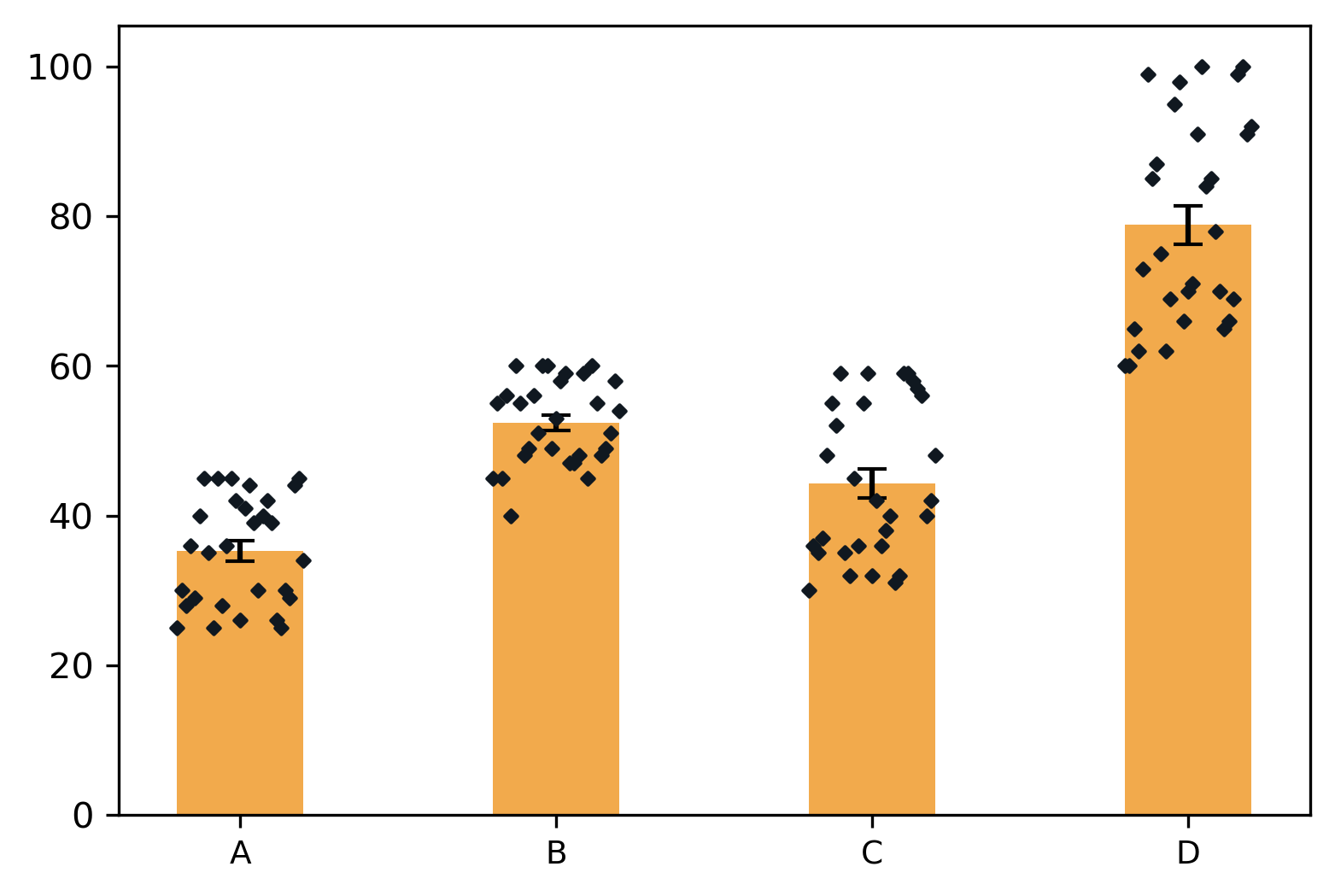Change the bar width,

``````# change shape of dot
# see available shapes at https://matplotlib.org/3.1.1/api/markers_api.html
>>> visuz.stat.bardot(df=df, bw=0.6)
``````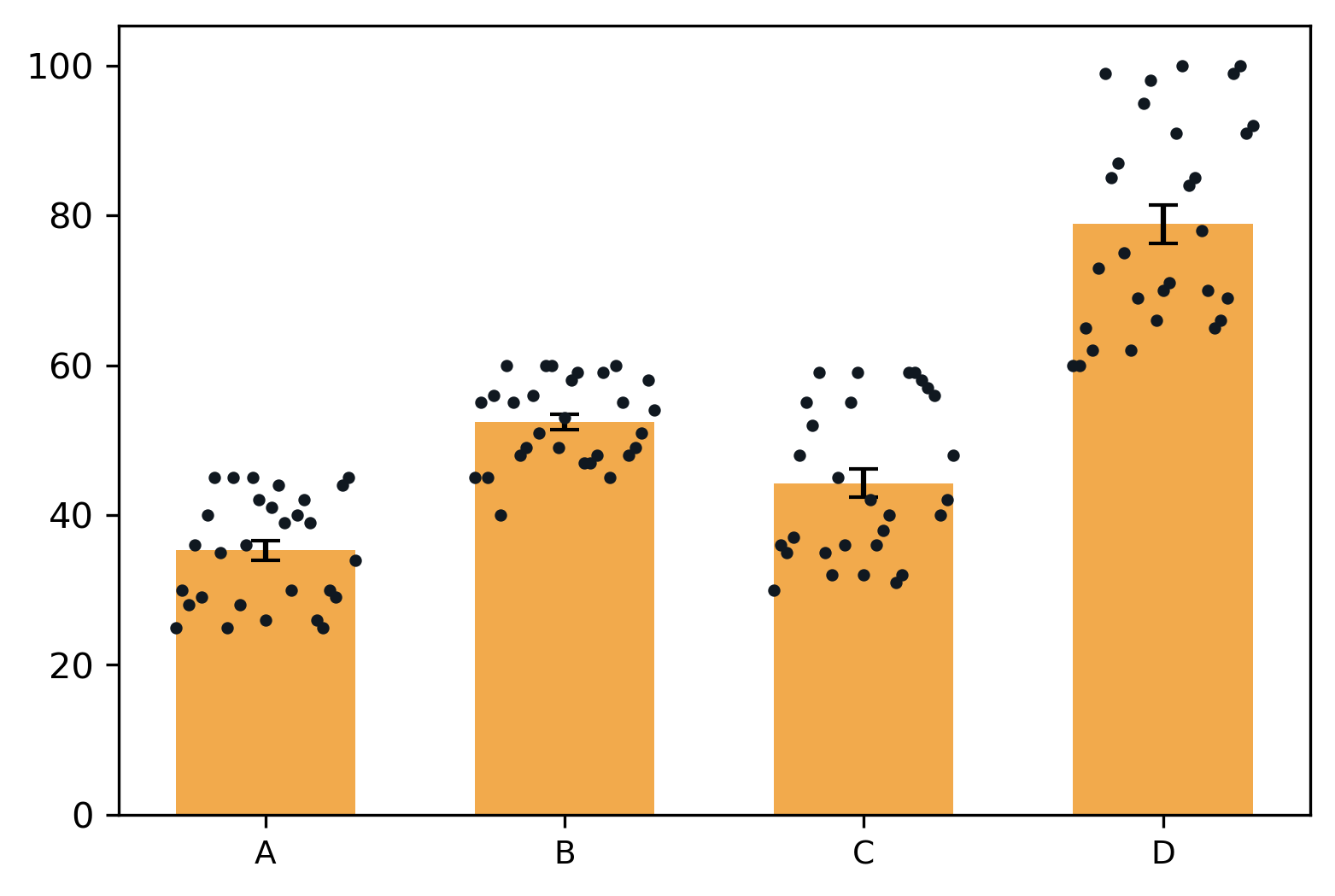Add the labels to the axis,

``````>>> visuz.stat.bardot(df=df, axxlabel='X', axylabel='Y')
``````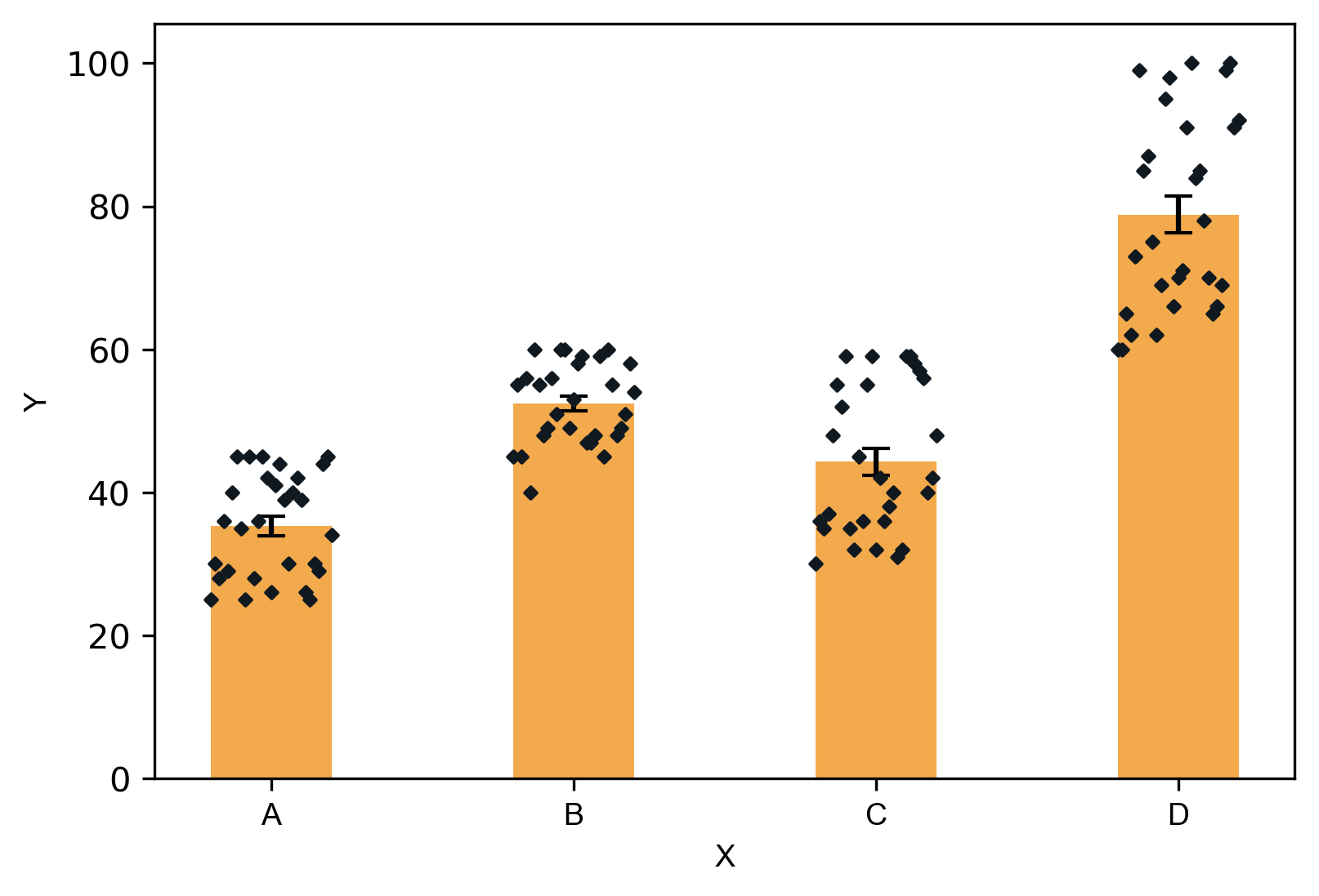In addition to these parameters, the parameters for figure type (`figtype`), figure dimension (`dim`), Y axis ticks range (`ylm`), axis labels (`axxlabel`, `axylabel`),
axis labels font size and name (`axlabelfontsize`, `axlabelfontname`), resolution (`r`), and axis tick labels font size and font name (`axtickfontsize`, `axtickfontname`) can be provided.

Check detailed usage

Last updated: April 9, 2020